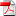# t and stable multivariate kernel density estimation by fast sum updating - N . Langrené, X. Warin

18
Jun

Kernel density estimation and kernel regression are powerful but computationally expensive techniques: a direct evaluation of kernel density estimates at M evaluation points given N input sample points requires a quadratic O(MN) operations, which is prohibitive for large scale problems. For this reason, approximate methods such
as binning with Fast Fourier Transform or the Fast Gauss Transform have been proposed to speed up kernel density estimation. Among these fast methods, the Fast Sum Updating approach is an attractive alternative, as it is an exact method and its speed is independent of the input sample and the bandwidth. Unfortunately, this
method, based on data sorting, has for the most part been limited to the univariate case. In this paper, we revisit the fast sum updating approach and extend it in several ways. Our main contribution is to extend it to the general multivariate case for general input data and rectilinear evaluation grid. Other contributions include its extension
to a wider class of kernels, including the triangular, cosine and Silverman kernels, its combination with parsimonious additive multivariate kernels, and its combination with a fast approximate k-nearest-neighbors bandwidth for multivariate datasets. Our numerical tests of multivariate regression and density estimation con rm the speed, accuracy and stability of the method.

Keywords: adaptive bandwidth; fast k-nearest-neighbors; fast kernel density estimation; fast kernel regression; fast kernel summation; balloon bandwidth; multivariate partition; fast convolution

•RR-FIME-18-04